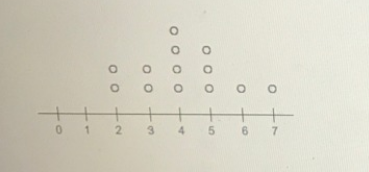# The dot plot represents the scores of a group of students on an English

The dot plot represents the scores of a group of students on an English test if the dot plot is converted to a box plot the first quartile would be drawn at (2, 3, 4, 5) and the median would be drawn at (2, 3, 4, 5)